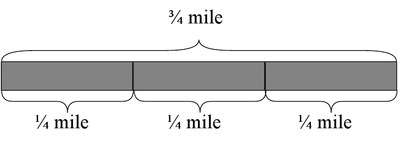# Running to School, Variation 2

Alignments to Content Standards: 6.NS.A.1

The distance between Rosa’s house and her school is $\frac34$ mile. She ran $\frac14$ mile. What fraction of the way to school did she run?

## IM Commentary

This task builds on a fifth grade fraction multiplication task, “5.NF Running to School, Variation 1.” This task uses the identical context, but asks the corresponding “Number of Groups Unknown” division problem. See “6.NS Running to School, Variation 3” for the “Group Size Unknown” version.

## Solutions

Solution: Solution

We know that the distance between Rosa’s house and her school is $\frac34$ of a mile.We also know that Rosa ran $\frac14$ of a mile.From the first picture we see that Rosa’s trip to school is broken up into three equal pieces, each representing $\frac14$ of a mile, which is $\frac13$ of her trip to school. Thus, Rosa ran $\frac13$ of the way to her school.

Solution: Taking a computational approach

This question is equivalent to asking, "What fraction of $\frac34$ mile is $\frac14$ mile?" We can write this symbolically as $$? \times \frac34 = \frac14$$ which is equivalent to the division problem $$\frac14 \div \frac34=?$$ Since $$\frac14 \div \frac34 = \frac14 \times \frac43 = \frac{4}{12} = \frac13$$ we see we get the same answer as if we did reasoning about the context in the previous solution.

Rosa ran $\frac13$ of the way to school.